## Geometric Series

A geometric series is the sum of the terms in a geometric sequence. If the sequence has a definite number of terms, the simple formula for the sum is

Formula 3:This form of the formula is used when the number of terms ( n), the first term ( a 1), and the common ratio ( r) are known.

Another formula for the sum of a geometric sequence is

Formula 4: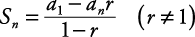This form requires the first term ( a 1), the last term ( a n ), and the common ratio ( r) but does not require the number of terms ( n).

##### Example 1

Find the sum of the first five terms of the geometric sequence in which a 1 = 3 and r = –2.

a 1 = 3, r = –2, n = 5

Use Formula 4:##### Example 2

Find the sum of the geometric sequence for which.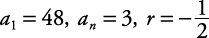Use Formula 4: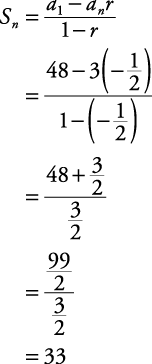##### Example 3

Find a 1 in each geometric series described.

1. S n = 244, r = –3, n = 5

2. S n = 15.75, r = 0.5, a n = 0.25

1. S n = 244, r = –3, n = 5

Use Formula 3: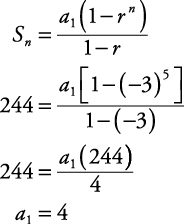2. S n = 15.75, r = 0.5, a n = 0.25

Use Formula 4: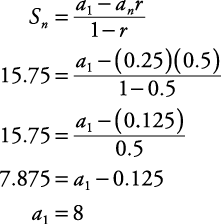Formula 5:

If a geometric series is infinite (that is, endless) and –1 < r < 1, then the formula for its sum becomesIf r > 1 or if r < –1, then the infinite series does not have a sum.

##### Example 4

Find the sum of each of the following geometric series.

1. 25 + 20 + 16 + 12.8 + …

2. 3 – 9 + 27 – 81 + …

1. 25 + 20 + 16 + 12.8 + …

First find r.Since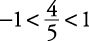, this infinite geometric series has a sum.

Use Formula 5.2. 3 – 9 + 27 – 81 + …

First find r.Since –3 < –1, this geometric series does not have a sum.

Back to Top
A18ACD436D5A3997E3DA2573E3FD792A